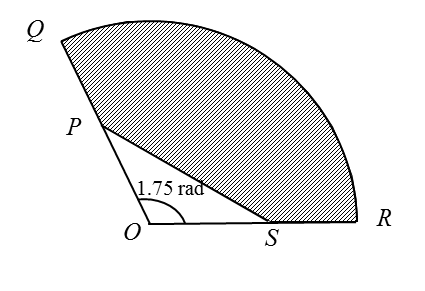# 1.4.1 Circular Measure Short Questions (Question 1, 2 & 3)

Question 1:The figure shows the sector OCB of radius 13 cm at the centre O. The length of the arc CB = 5.2 cm. Find
(b) the perimeter of the shaded region.

Solution:
(a)

(b)

Perimeter of the shaded region = 5.07 + 1.03 + 5.2 = 11.3 cm.

Question 2:The figure shows the sector AOB of a circle, centre O and radius 5 cm. The length of the arc AB is 6 cm. Find the area of:
(a) the sector AOB,

Solution:
(a) Arc AB = 6cm
s = θ
6 = 5 θ

(b)

Question 3:
Diagram below shows a sector QOR of a circle with centre O.It is given that PS = 8 cm and QP = PO= OS = SR = 5 cm.
Find
(a) the length, in cm, of the arc QR,
(b) the area, in cm2, of the shaded region.

Solution:
(a) Length of arc QR = θ = 10 (1.75) = 17.5 cm

(b)
1.